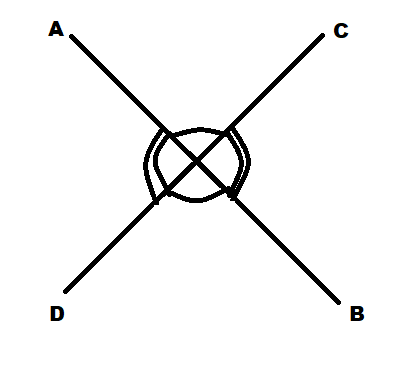QuestionAnswers

# If two lines intersect such that four vertical angles are equal then each angle isA) $45^\circ$B) $100^\circ$C) $180^\circ$D) $90^\circ$Verified
129k+ views
Hint: First of all let each angle be x and then apply the angle sum property of to find the value of x. Since each angle is equal therefore the value of all four angles will be x.Angle sum property is given by:
${\text{A + B + C + D}} = {360^\circ }$

Complete step by step solution:
Let each angle be $x$.
Then according to the angle sum property of vertical angles, the sum of all four angles is equal to ${360^ \circ }$.
i.e, if A,B,C,D are four vertical angles of two intersecting lines then according to the angle sum property:
$A+B+C+D=360^\circ$
Now here since all four angles are equal therefore,
${\text{A = B = C = D = x}}$
Now , putting in the value of A,B,C,D in the above equation we get:
$x + x + x + x = {360^ \circ }$
On simplifying the above equation,
$\Rightarrow 4x = {360^ \circ }$
On further calculations,
$\Rightarrow x = {90^ \circ }$

$\therefore$ Each angle is equal to $90^\circ$. Hence, the correct option is option (D).

Note: When two lines intersect then the sum of angles between them is equal to ${360^ \circ }$ by angle sum property. Just observe the image and relate the equal angles on both sides. It will be easy to understand.